Omer Goldberg

# Uniswap V3 TWAP: Assessing TWAP Market Risk## Abstract

Time-weighted average price (TWAP) oracles have become indispensable in the decentralized finance (DeFi) sector, providing crucial price data for various applications. However, despite their adoption, the potential for manipulation of TWAP oracles poses a significant threat to the security and stability of DeFi protocols. The robustness, resilience, and price accuracy of TWAP oracles highly depend on market conditions, pool liquidity, and liquidity distribution per ticks, thus exposing them to market risk and liquidity fluctuations.

This paper assesses the likelihood and feasibility of manipulating Uniswap's V3 TWAP oracles, focusing on the worst-case scenario for low liquidity assets. To this end, we introduce the Chaos Labs TWAP Market Risk application, which leverages pool data, including liquidity depth and exhaustion prices, to quantify the real-time manipulation risk across all V3 pools and deployments.

Moreover, this study proposes strategies for TWAP Oracle consumers to mitigate the manipulation risks and explores the potential benefits of adopting median oracles as a viable alternative.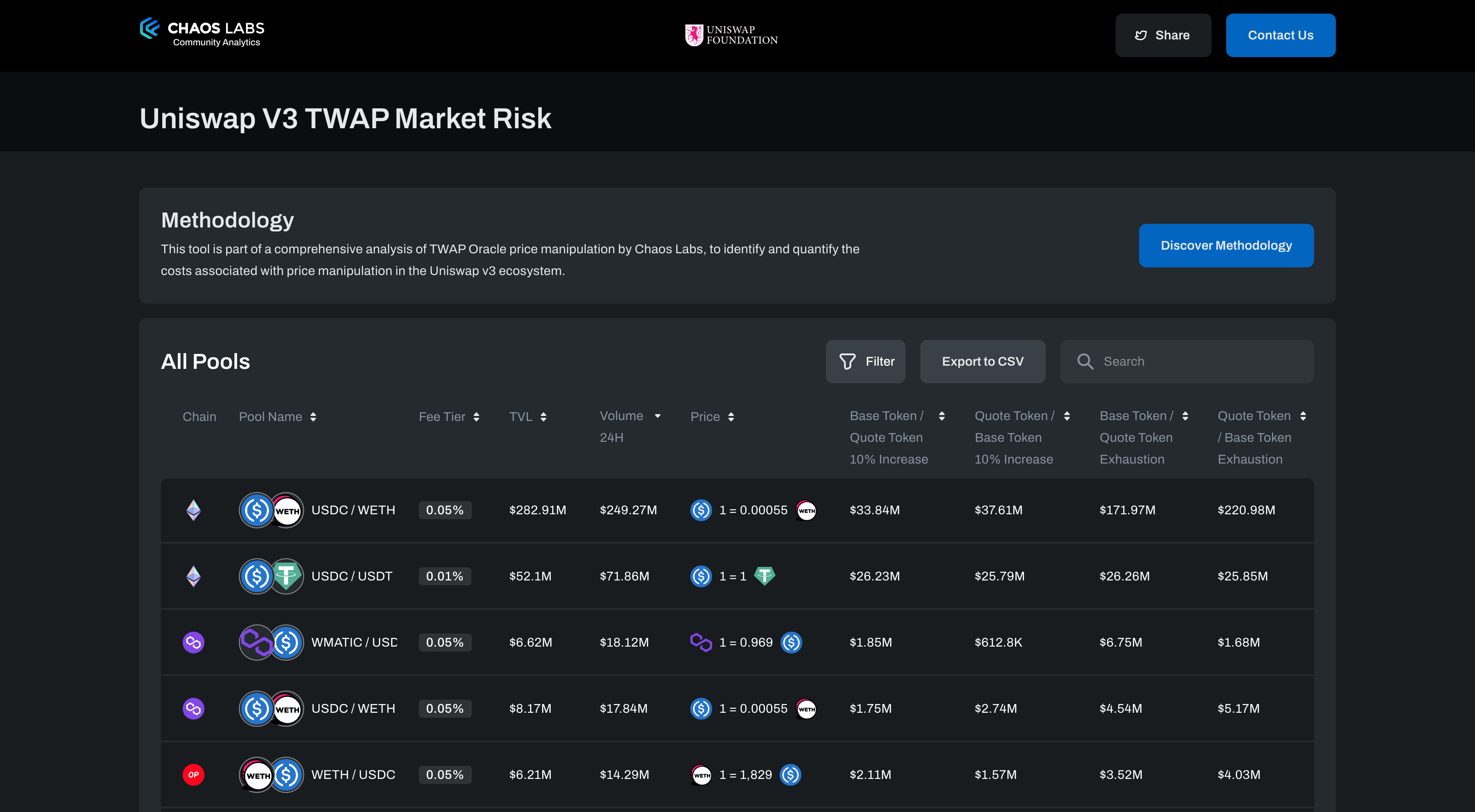## Why is Oracle Manipulation an attractive exploit vector for attackers?

The manipulation of TWAP oracles can have severe consequences for decentralized finance (DeFi) protocols, leading to potential economic exploits and financial losses. Lending and derivative protocols, for example, require accurate on-chain price information to calculate the market price of assets and determine the value of collateral and debt for loans issued by the protocol. In this context, an attacker may attempt to manipulate the TWAP oracle to overvalue their collateral, leading to undercollateralized loans and bad debt for the lending protocol, which benefits the attacker. Let’s look at two real-life attacks that exploited this attack vector.

### Moola Market Oracle Manipulation

Moola Market is a non-custodial lending protocol on the Celo blockchain that aims to democratize access to borrow and lend markets. The protocol was hacked on October 18th, 2022, using a simple, manual price manipulation exploit. The attacker began with initial funding of 243k $CELO and used 60k$CELO to borrow 1.8M $MOO tokens, which were then used as collateral to borrow against other assets. The remaining 183k$CELO was used to artificially inflate the price of $MOO collateral on Ubeswap, enabling the attacker to borrow the remaining assets on the protocol and drain all of its liquidity, resulting in a loss of approximately$9 million.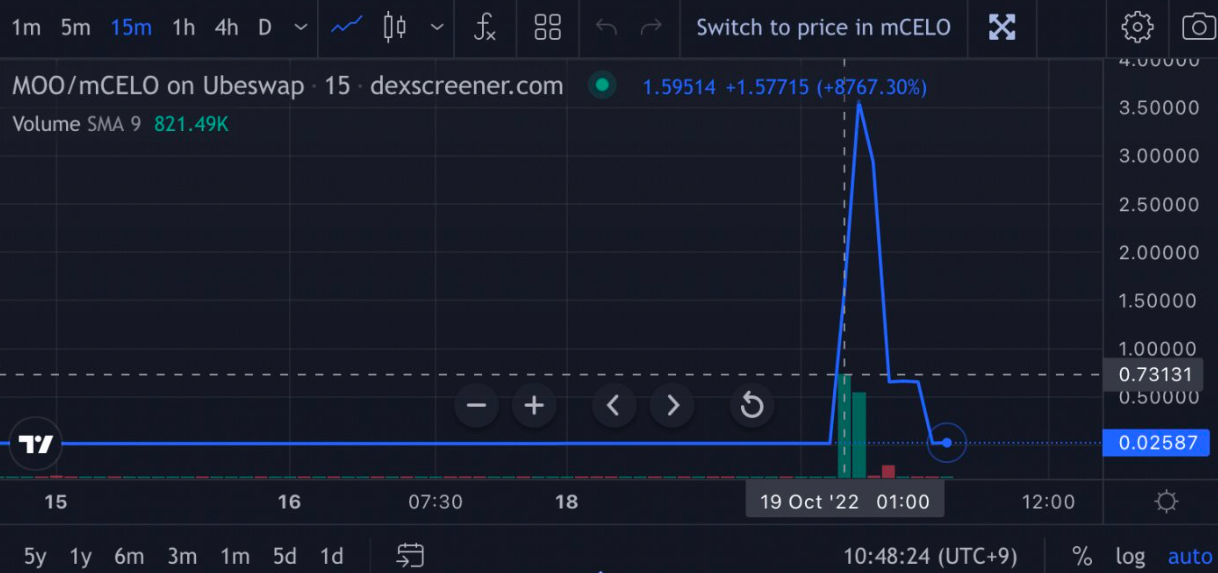### Mango Market Oracle Manipulation

Mango Markets is a decentralized perpetual exchange built on the Solana blockchain. It allows users to trade futures, perpetual swaps, and options with high leverage, low fees, and fast settlement times. The platform is designed to be non-custodial, meaning users retain control of their funds and can manage their own risk.

Here is a summary of the Mango Markets attack in a step-by-step format:

1. Account A was funded with $5M USDC. 2. Account A created a sell order for 483M MNGO units. 3. Account B was funded with$5M USDC and bid on Account A's sell order at an average price of $0.0382 per unit. 4. Account B pumped the MNGO spot markets to approximately$0.91.
5. Account B's unrealized profit was $421M after the MNGO spot markets reached$0.91.
6. Account B borrowed $116M worth of tokens and drained Mango Markets. 7. MNGO crashed to$0.02 after the exploit.
8. No liquidity was left to settle/close, leaving Account A's original short position worth 12M unsettled. The attacker successfully implemented a price pump strategy, which resulted in the complete depletion of Mango Markets' assets. This event can be characterized as a classic example of a price manipulation attack.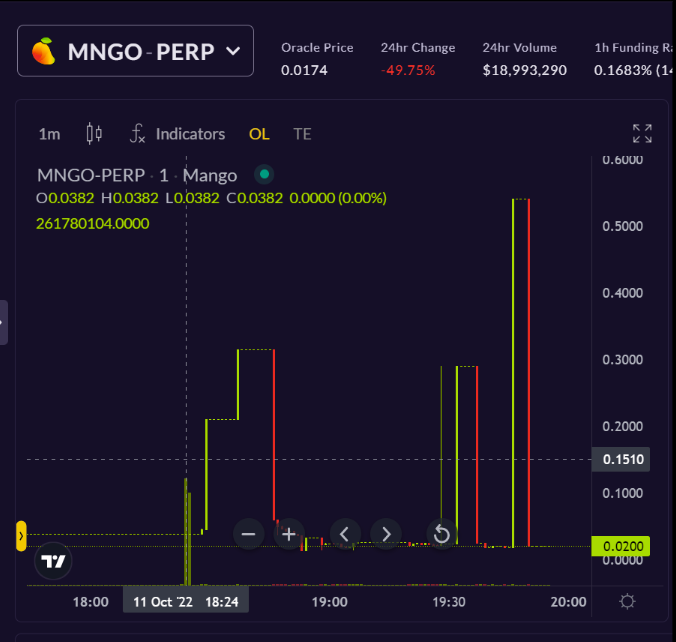Credit: Joshua Lim exploit thread ## Uniswap's V3Geometric TWAP Oracle The oracle's methodology for calculating the TWAP involves taking the geometric mean of an asset's spot price over a designated time window. Typically, most DeFi applications consume TWAP prices by specifying a time window of 30 minutes. This time window is deliberately chosen to minimize the impact of price manipulation and offers a more precise reflection of the asset's actual value. This article assumes that an attacker is attempting to manipulate a price derived from a 30-minute time frame. ## Calculating an average for mined Ethereum blocks in a 30-minute timeframe In the context of an economic exploit aimed at manipulating prices, an attacker must control a certain percentage of blocks within the desired 30-minute timeframe. However, the mining speed of blocks can vary across different blockchain networks, making it necessary to consider these factors when assessing the feasibility and potential impact of such attacks. Per Ethereum documentation, blocks are proposed by validators selected at random, and the block production time has been observed to range from 12 to 13 seconds. Assuming an average block production time of 12 seconds, we can calculate that approximately 5 blocks are mined per minute. Consequently, Uniswap's 30-minute TWAP encompasses approximately 150 blocks. However, historical data suggests that blocks can sometimes take up to 13 seconds to process. To increase the precision of our calculations, we will assume a block production time of 12.5 seconds, resulting in an estimated number of blocks within a 30-minute timeframe on Ethereum of approximately $n=144$. $n=144$, must be manipulated. This requires an attack to move the spot price to a target price, q, where $q$ is based on the number of blocks, m; the attacker can manipulate the spot price where $m \le n\approx144$. $q$ can be calculated using the following equation: $q = \sqrt[m]{\frac{TWAP^n}{p^{(n-m)}}}$ ## Feasibility of Manipulation To assess the feasibility of manipulating Uniswap's TWAP oracles, we first examine the capital required to move the spot price of an asset to the desired TWAP price. We then consider the probability of an attacker controlling a significant number of consecutive blocks on the Ethereum blockchain, which would be necessary to maintain the manipulated price over the time window. Let’s breakdown the process into where an attacker profits from TWAP manipulation: • The attacker selects a new TWAP price $q$, for an asset • The attacker moves the asset price from $p$ to $q$ • Price movement is done via swapping into the target liquidity pool • $q$ calculated based on TWAP, $p$, and $m$ • $q$ can be greater than or lesser than $p$ • Lending protocol • $q > p$ allows the attacker to deposit overpriced assets as collateral and borrow more than the true value of the collateral • $q < p$ allows the attacker to borrow more of the underpriced asset than their collateral is worth • Perp Exchanges • $q > p$ allows an attacker to profit from a long position • $q < p$ allows an attacker to profit from a short position ## Calculating Target Price The first step to assessing the general viability of these attacks - especially with low liquidity assets - is to calculate $q$ and the capital required (i.e., swap size) to change an asset's price from $p$ to $q$. The manipulated TWAP price can be understood as some constant,$k$, multiplied by $p$ where $k > 1$ when increasing the spot price and $k < 1$ when decreasing the spot price $TWAP = k*p$ With this, we can simplify our calculation of $q$: $q = \sqrt[m]{\frac{(kp)^n}{p^{(n-m)}}}$ $q = \sqrt[m]{\frac{k^np^n}{p^{(n-m)}}}$ $q = \sqrt[m]{k^np^m}$ $q = p\sqrt[m]{k^n}$ Finally, we isolate the constant term: $\frac{q}{p} = \sqrt[m]{k^n}$ This is similar to saying k is some ratio of TWAP and $p$: $k = \frac{TWAP}{p}$ As we’ve estimated $n = 144$, we can look at various values of m to see how large a price movement is needed to manipulate an asset's TWAP.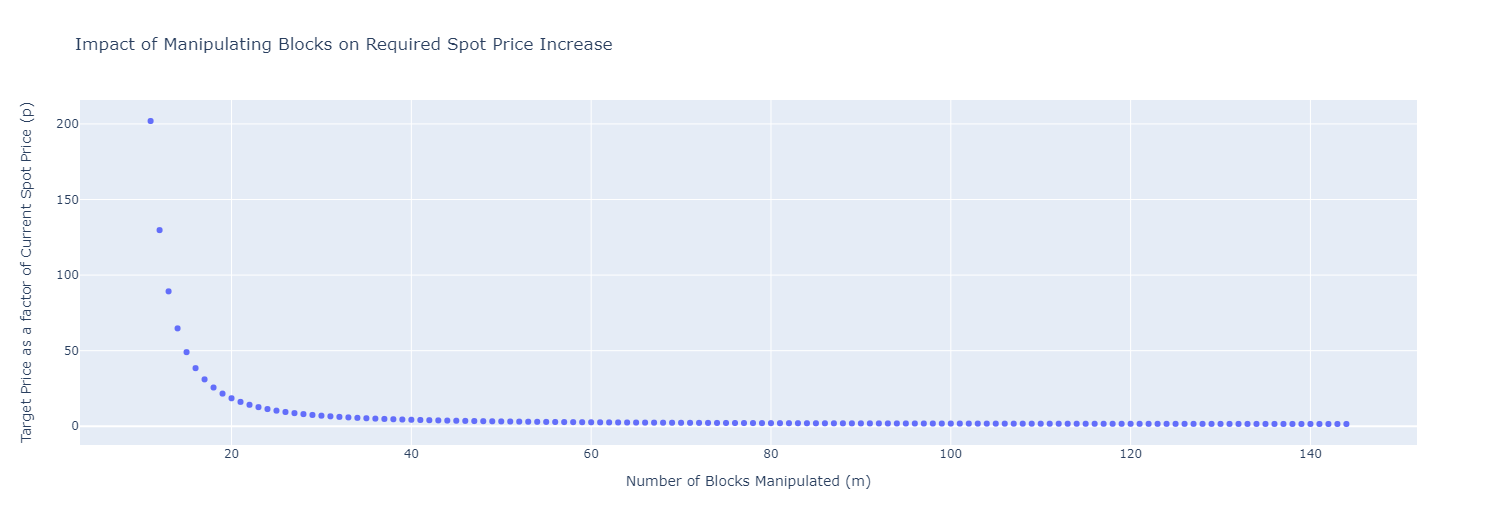As $m$ approaches $n$, the ratio $q/p$, our y-axis, approaches $k$. This is because $q$ approaches TWAP as $m$ approaches $n$. This can be seen by substituting $m = n$ in our earlier equation: $\frac{q}{p} = \sqrt[n]{k^n}$ $\frac{q}{p} = k$ We already know k is the ratio of TWAP over p, so with a quick substitution: $\frac{q}{p} = \frac{TWAP}{p}$ $q = TWAP$ The optimal scenario for an attacker consists of adjusting the spot price of an asset to align with their desired TWAP value, subsequently inhibiting any further transactions throughout the duration of the TWAP window (e.g., the subsequent 144 blocks in this instance). Although this situation is rather implausible, it is essential to examine the worst-case scenario. If this scenario proves impractical (i.e., not lucrative or necessitating an exorbitant capital requirement), further evaluation of manipulation concerns becomes redundant. Subsequent sections of this paper will explore the feasible dimensions of $m$ and $n$ within Ethereum and other blockchains where Uniswap is employed. Given the liquidity provider's (LP) liquidity and the corresponding price range, it is possible to ascertain the quantity ofLOOKS needed to elevate its price.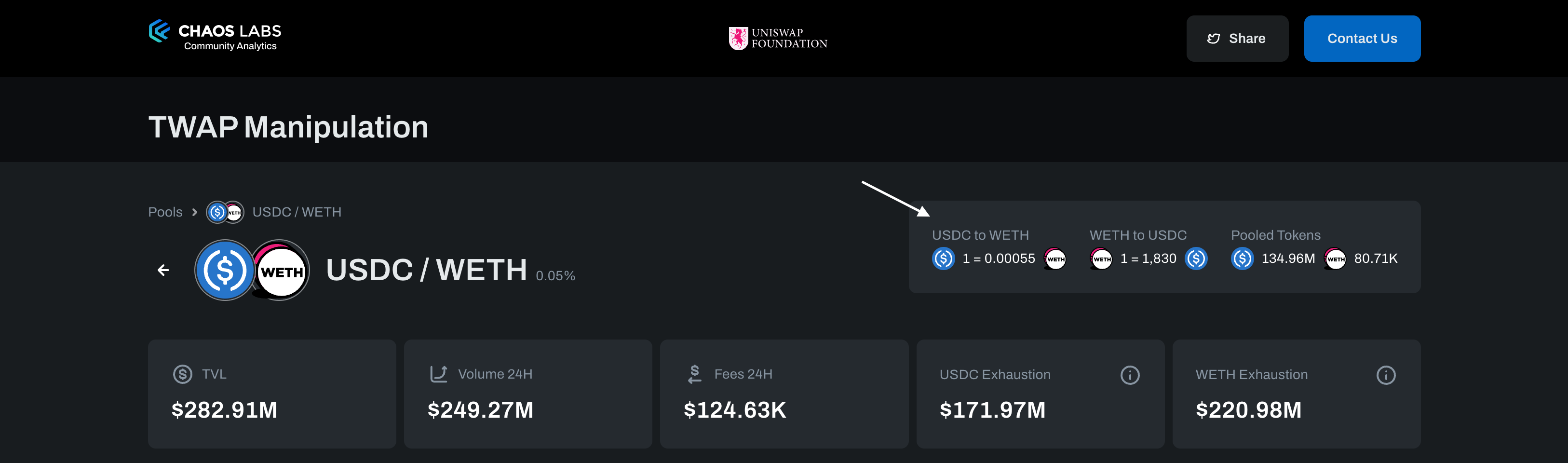For further intuition, here is a code snippet that utilizes the Uniswap Quoter Contract and querying quoteExactOutputSingle, to determine the exact amount of ETH that must be sold to acquire the requisite $LOOKS. When taking a look at the$ETH required, an interesting fact emerges: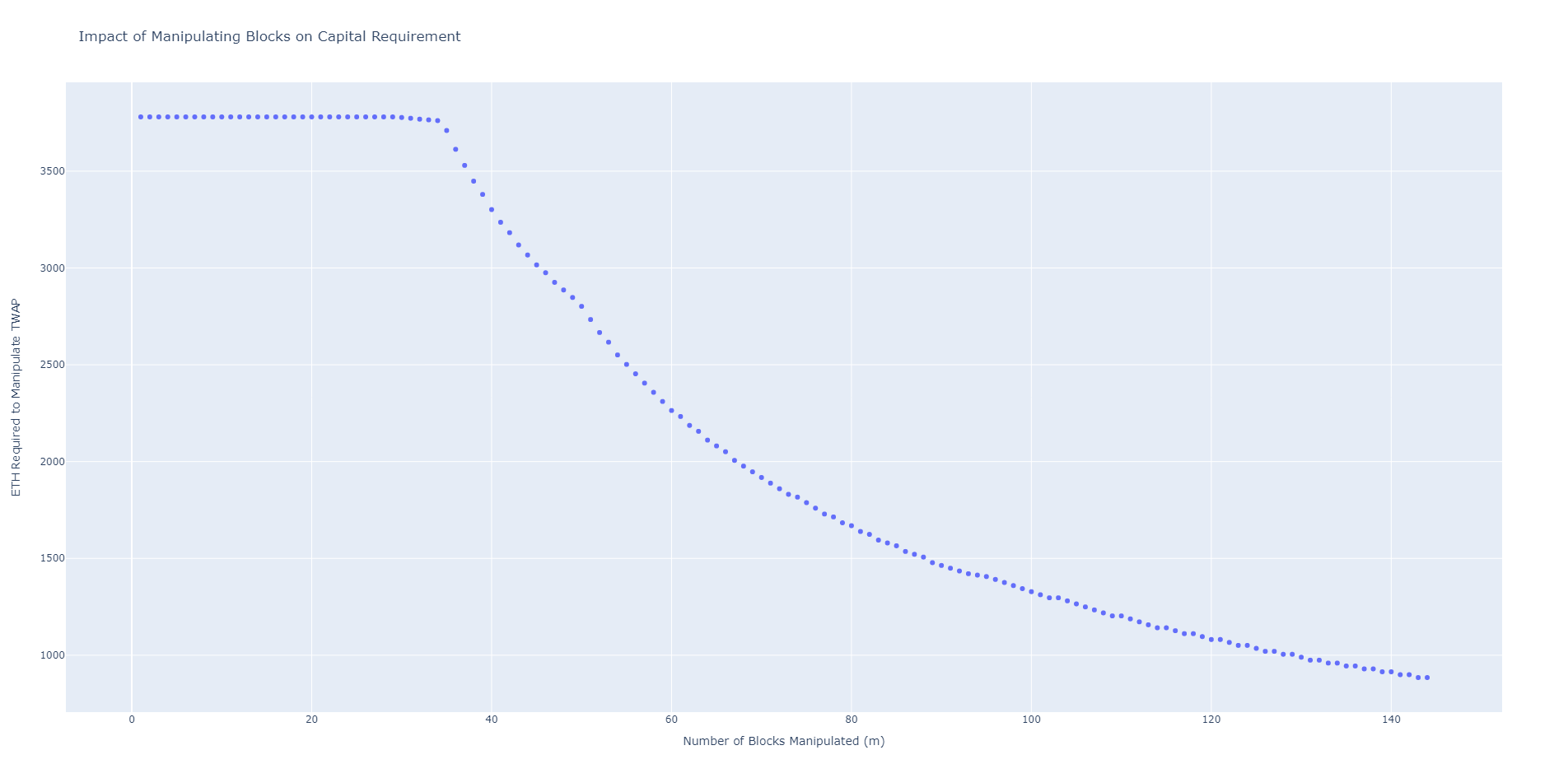Until the attacker can manipulate 30 or more blocks, approximately 3,781 ETH (5.9 million USD) must shift the spot price from $p$ to $q$. This figure represents the ETH required to deplete the $LOOKS pool. In Uni v2, a pool expressed the token price from 0 to infinity, rendering the exhaustion of a single asset in the pool nearly impossible. However, with concentrated liquidity, asset exhaustion within a pool is now achievable. Once the pool is depleted, it becomes simple to add liquidity at the desired price range and complete the asset's spot price manipulation. The exhaustion cost for a pool should be considered when contemplating risk mitigation strategies. In the worst-case scenario, an attacker would need approximately 885 ETH (1.4 million USD) to manipulate the spot price. The amounts of 1.4 million USD and 5.9 million USD are considerably large compared to the roughly 3.8 million USD total value locked (TVL) of the pool. The exhaustion cost for any pool can be observed via the Chaos Labs TWAP Market Risk detail page as follows: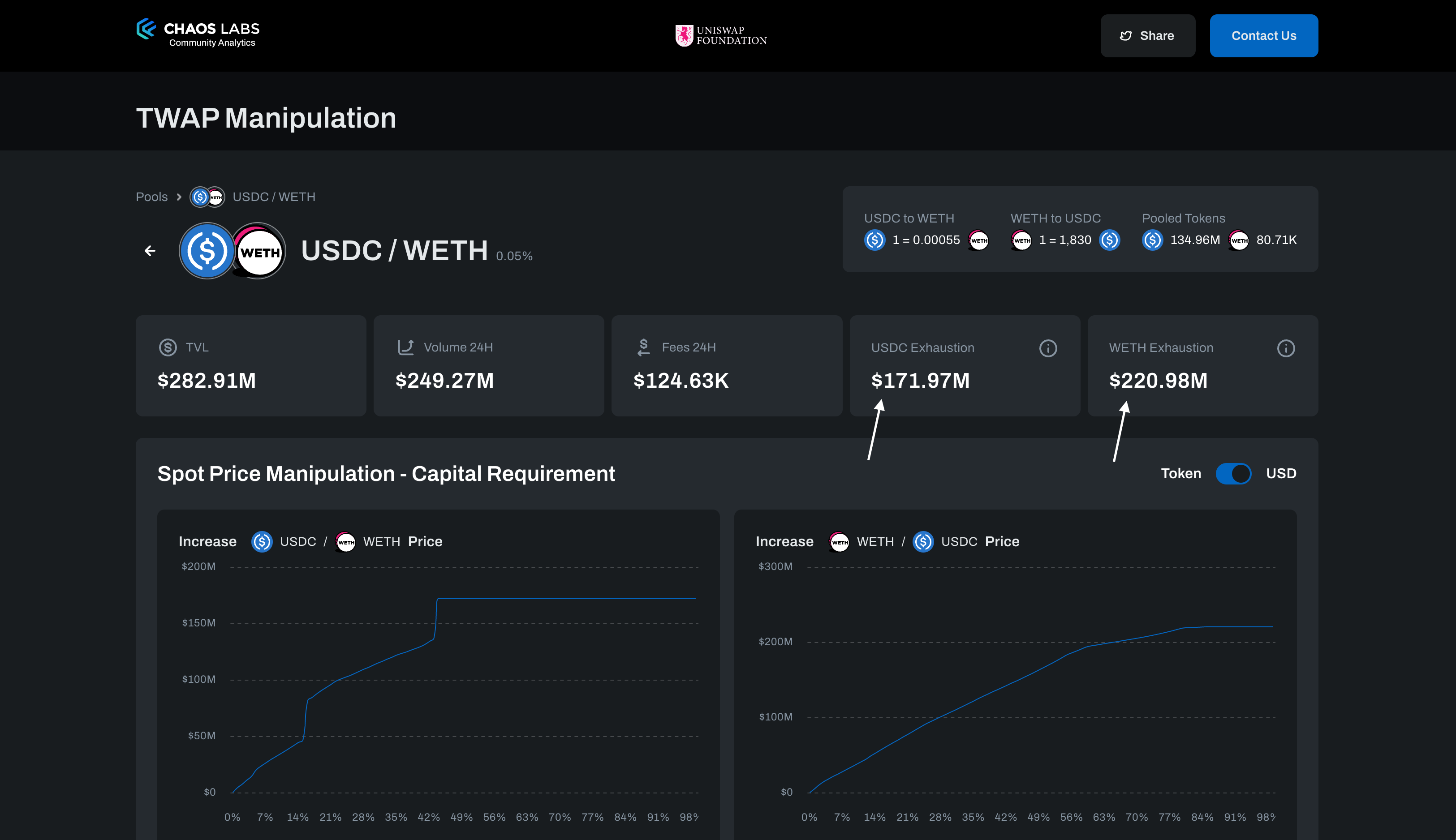The cost of executing a successful attack displays a considerable range, with a lower bound of 1.4 million USD. However, it is important to note that this figure requires the attacker to maintain control over a consecutive sequence of 144 blocks. Conversely, an upper bound of 5.9 million USD is near twice the total value locked (TVL) of the ETH/LOOKS pool. This higher figure assumes that the attacker cannot exercise control over a significant number of blocks and sets a relatively high threshold of 30 blocks. It is worth noting that the optimal threshold may vary between different pools. Therefore, a robust methodology that can account for the likelihood of block manipulation by attackers is imperative for accurately estimating the cost of executing an attack. Thus, a method to factor in the probability of attackers manipulating blocks is necessary. ## Generalizing Capital Requirements The capital requirement for manipulating a TWAP oracle varies greatly depending on the liquidity distribution within a pool. Narrower liquidity is cheaper to manipulate than its wider counterpart. Also, there is no single exhaustion cost for a pool’s assets. The ETH/LOOKS example used here looks at the number of ETH required to buy all the LOOKS in the pool. The number of LOOKS required to buy all the ETH is not necessarily the same. We can see that by looking at the current pool liquidity distribution: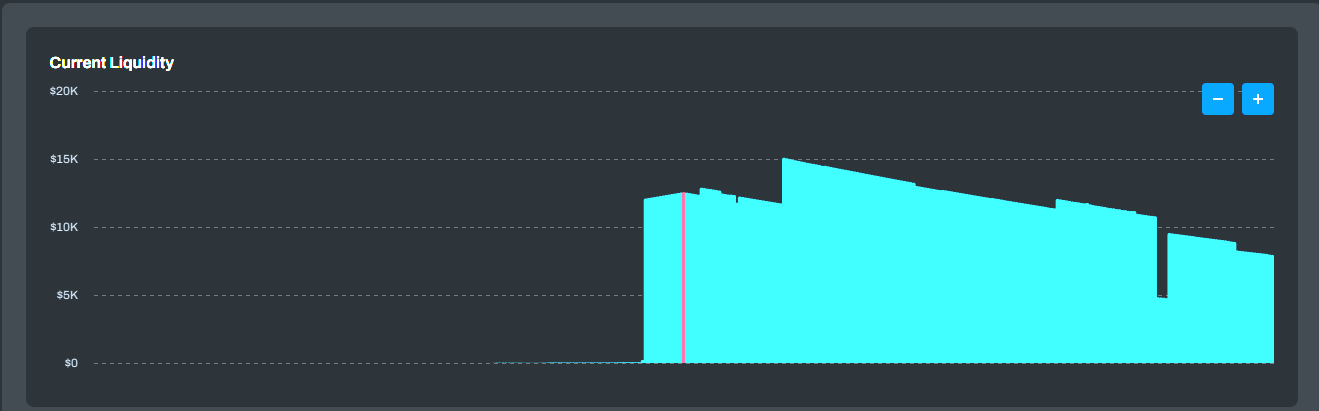The pool liquidity is asymmetrical. Increasing the LOOKS / WETH price requires more capital than decreasing the WETH / LOOKS price. This makes using the pool TVL misleading. An attacker would select the lowest single asset liquidity pool, not the pool with the lowest TVL. The process is fairly manual, i.e., the assets needed to move a pool's spot price depend on the pool's tick-by-tick liquidity. To assist Uniswap users who have Oracle manipulation concerns, Chaos Labs has implemented a Uniswap V3 TWAP Market Risk application. This interactive dashboard summarizes the capital requirement for moving an asset’s spot price: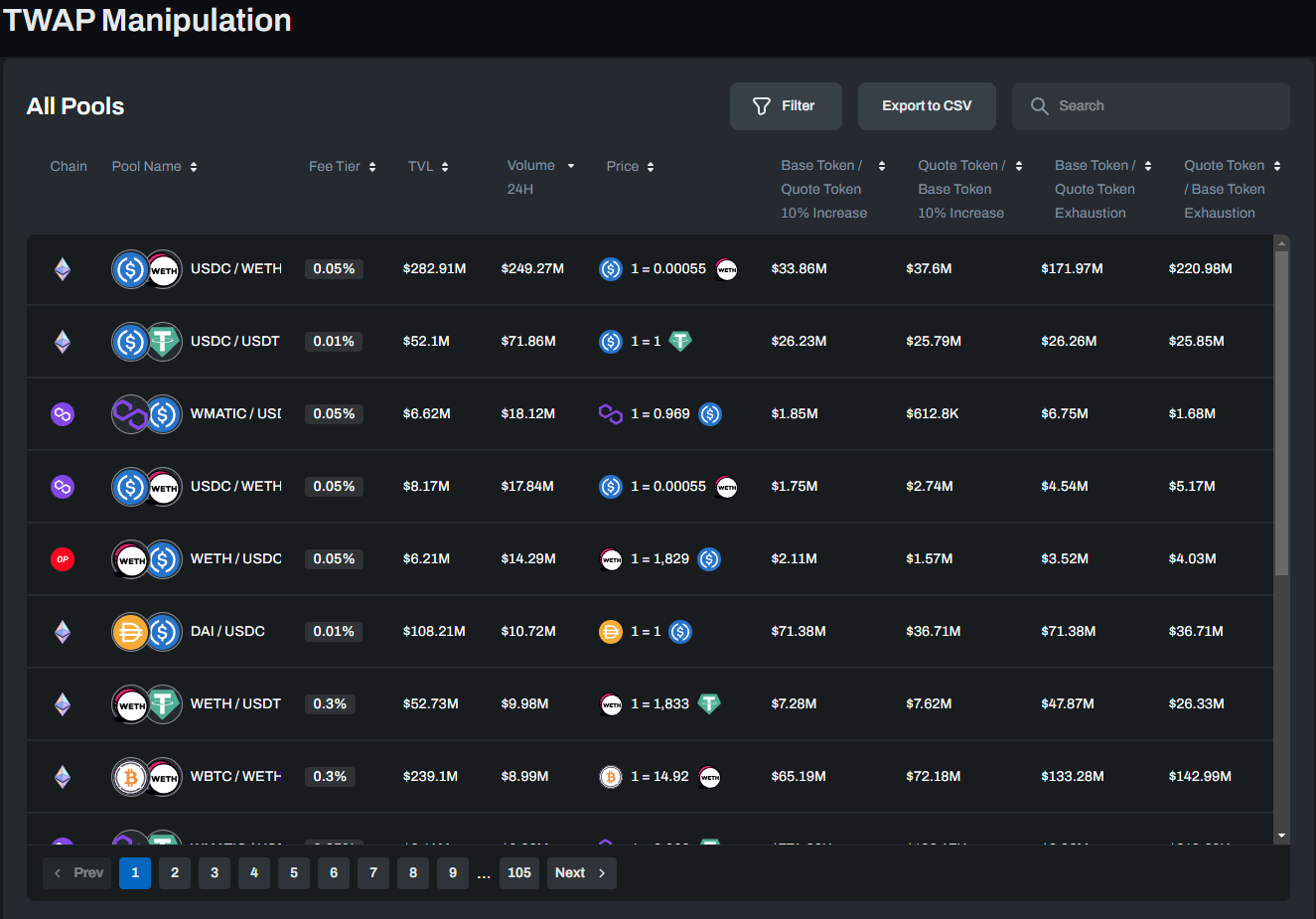Within a single pool, we can see the requirement of moving each asset is not identical:These images were taken at a different time (i.e., different TVL, asset prices, etc.) than the prior images on the capital requirement, so the numbers are not identical. The capital requirement for increasing the WETH / LOOKS price caps at$172.63k. The capital requirement for increasing the LOOKS / WETH price caps at $7.41M. This circles back to the uneven distribution of liquidity shown before. The pool has significantly more$LOOKS (~$3m) than WETH (~$101.3k). The $LOOKS liquidity is also wider than its WETH counterpart. This can be seen by the ratios of capital requirement to liquidity for each asset: $7,410,000 / 3,000,000 = 2.47$ $172,630 / 101,300 \approx 1.70$ We can also look at what percent price manipulation requires LP exhaustion: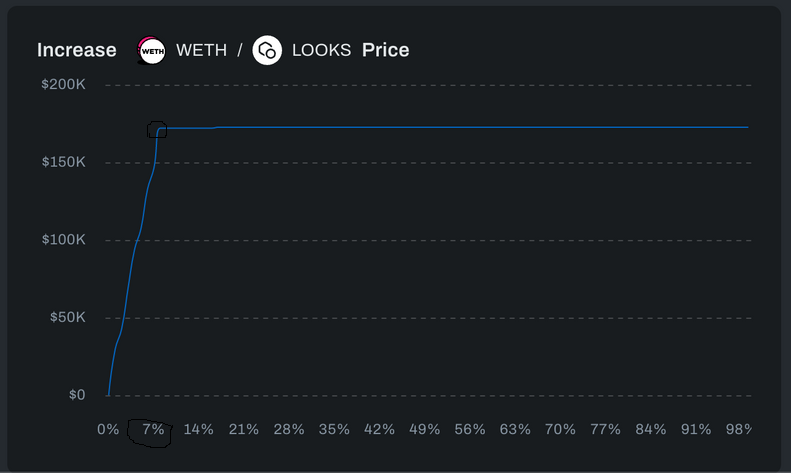The WETH / LOOKS pair caps out a bit past 7%. Until then, a roughly linear relationship exists between desired price manipulation and capital requirement. Now look at the LOOKS / WETH pair: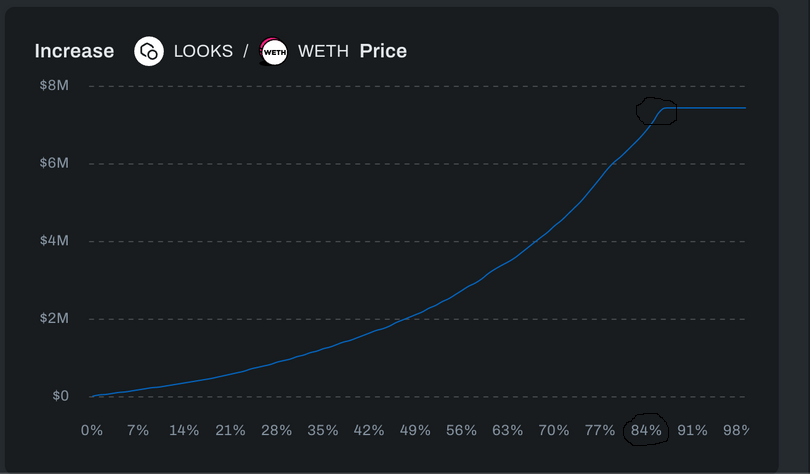It caps out at about 84%. Notice the non-linear curve from 0% to 84% as well. The amount and distribution of liquidity for each asset determine the difficulty in manipulating their price. You can see how the numbers change with respect to the number of blocks controlled by an attacker: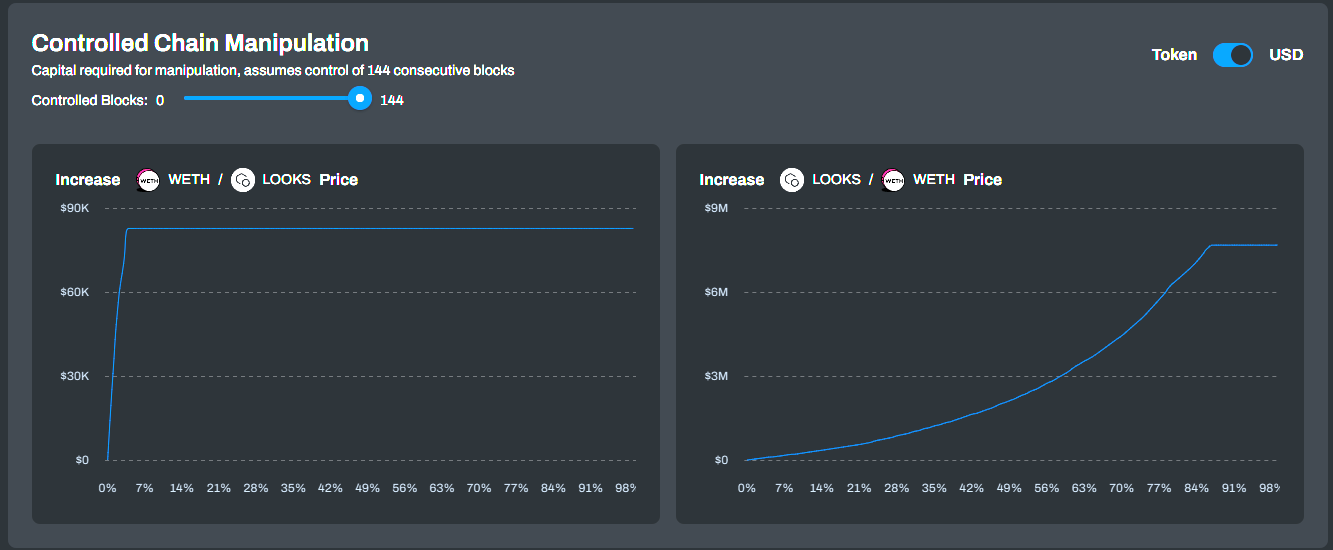You can change the number of blocks controlled and see the capital requirement for manipulating each asset. The above example shows an attacker controlling 144 consecutive blocks. Below is the capital requirement if they control 72 blocks instead: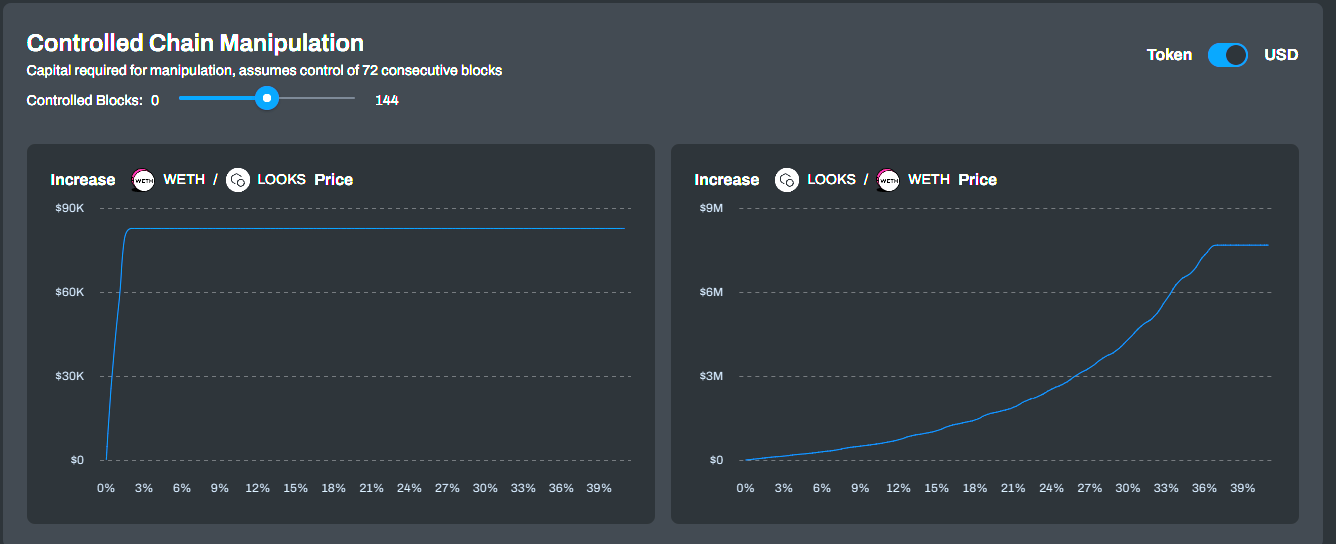This tool allows you to test and see the capital requirements of an attack for any number of blocks controlled. The next section will discuss the practicality of controlling $m$ consecutive blocks. With that information and this tool, you can choose an attack scenario (i.e., the number of blocks controlled) and see the USD swap size needed to manipulate the Oracle price. ## Block Manipulation The feasibility of a successful attack on a Uniswap pool is primarily determined by the capital required and the number of blocks the attacker must control. To calculate the capital required for an attack, we can use the exhaustion cost of the pool unless the attacker can control a significant number of blocks. The threshold for the number of blocks required to control depends on the liquidity distribution within the pool. In the case of the LOOKS/WETH pool, the threshold is approximately 30 blocks, as demonstrated in our TWAP market risk assessment. It is necessary to evaluate the feasibility of controlling 30 consecutive blocks in a 30-minute time frame. A validator chosen randomly produces each block, with the validator able to select the transactions processed in the block. Therefore, an attacker would have to operate a set of validators that could control 30 consecutive blocks. While selected validator nodes are chosen randomly, there are staking pools such as LiDo that control multiple nodes. Thus the probability of a pool proposing a block can be modeled as $\frac{V_p}{\sum{V}}$. $V_p$ is the number of nodes the pool controls, and $\sum{V}$ is the total number of active nodes. If we look at the probability of a pool proposing $m$ out of $n$ blocks, the equation would be: $P(X=m)=\begin{pmatrix}n \\ m\end{pmatrix}P(V_p)^m(1-P(V_p))^{(n-m)}$ With an expected value of $E(X)=P(V_p)*n$. Dune Analytics offers a comprehensive dashboard that displays the percentage of nodes controlled by various pools. As of writing this, the largest entity controls 31.27% of the nodes, with approximately 23.15% of the nodes remaining unidentified. It is essential to consider the share controlled by unidentified entities when assessing the risk of Sybil attacks. One possible attack scenario could involve the largest entity Sybiling, controlling the unidentified nodes, resulting in 54.42% of the nodes under the attacker's control. Based on our analysis, we anticipate the attacker to control roughly 78 out of every 144 blocks, given the concentration of nodes. For the ETH/LOOKS pool, this translates to a capital requirement of approximately 1,716 ETH (equivalent to 2.7 million USD), significantly lower than the exhaustion cost of the pool, which is 5.9 million USD. Notably, the vast majority of$LOOKS liquidity is concentrated within the ETH/LOOKS 0.3% fee pool, with only a nominal amount (~280k) present in the v2 LOOKS/ETH LP. As such, no significant source of liquidity is available on Uniswap to facilitate the "correction" of $LOOKS prices in the event of an attack. Additionally, it is unrealistic to expect arbitrage bots to hold liquid quantities of$LOOKS in their wallets, further compounding the liquidity constraints during an attack. While holders of $LOOKS may attempt to sell their holdings at inflated prices during a 30-minute attack, it is unlikely that such activity would occur on an automated basis similar to that of arbitrage bots.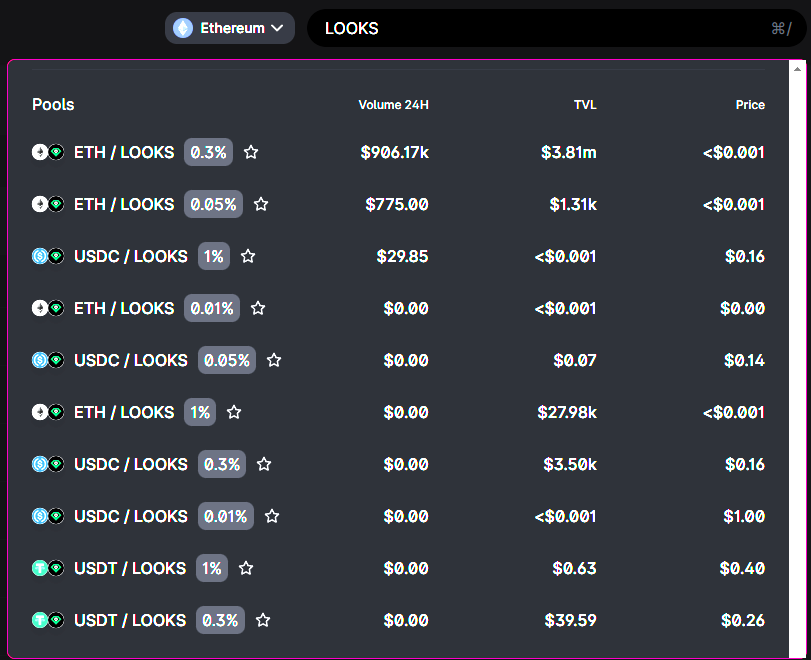Our earlier attack scenario had an entity controlling 54.42% of the validators. Let's look at the probabilities for that entity controlling consecutive blocks. We’ll be simulating the block assignments and estimating the probability accordingly.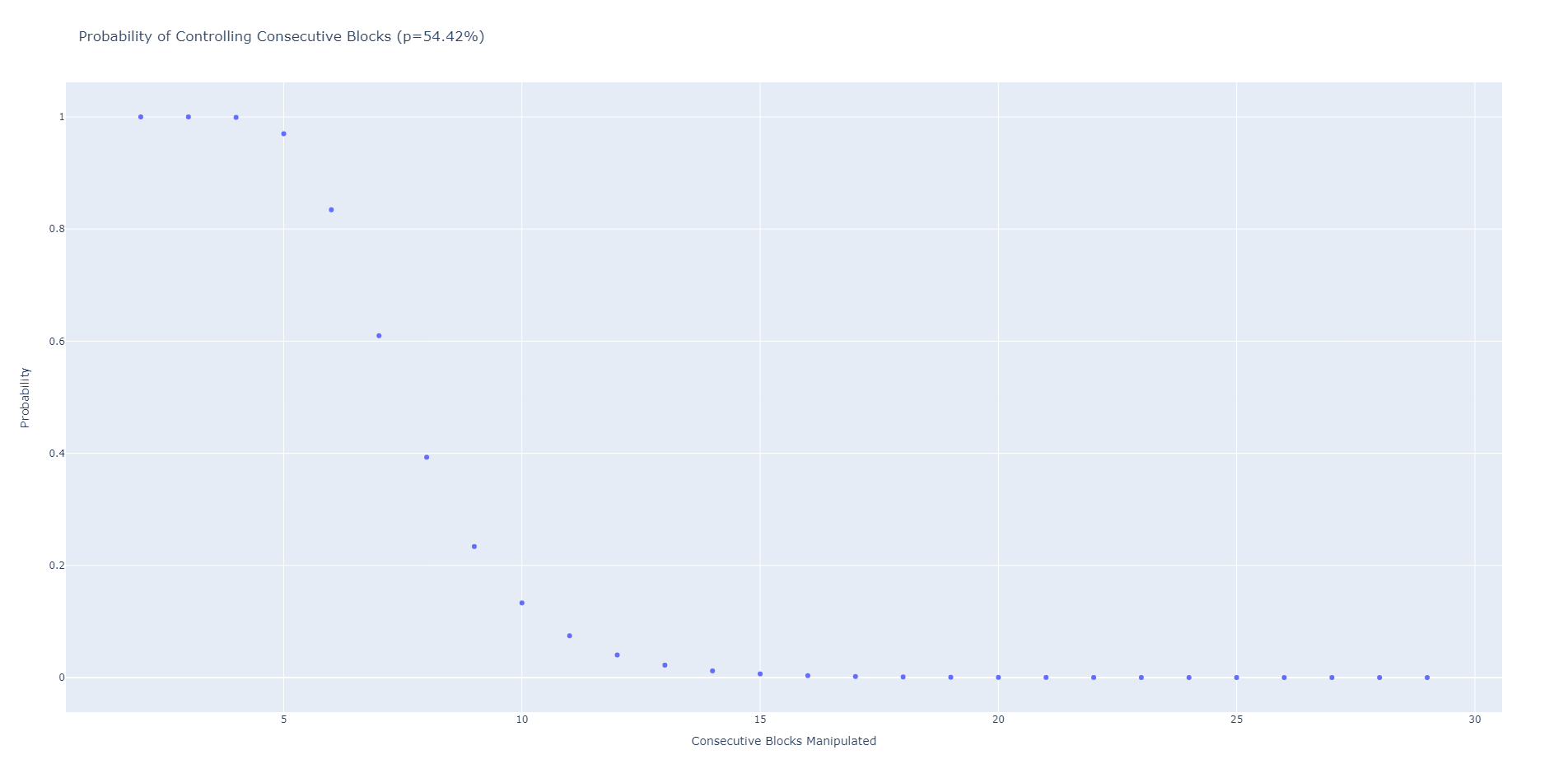The probability approaches and effectively hits 0 by 15 consecutive blocks. This is less than the ~30 blocks required not to exhaust the LOOKS / WETH LP of all its assets. Thus, assuming attacks will only occur during a set of consecutive manipulated blocks defaults back to the exhaustion cost calculated before. ## Alternative Chains While our calculations and examples have focused on Uniswap deployed on Ethereum, it is worth noting that Uniswap is also deployed on other chains, including Arbitrum, Celo, Optimism, and Polygon. The query function operates similarly on all these chains, with the primary difference being the PoS mechanism employed by each chain. It is crucial to consider whether these PoS mechanisms impact the feasibility of an attacker controlling a significant number of blocks. Below is a summary of the chains and their block PoS mechanisms: • Arbitrum: Uses a rollup architecture, which relies on PoS consensus to validate transactions. • Celo: Employs a hybrid PoS mechanism, where validators are selected based on a combination of stake and random selection. • Optimism: Uses a rollup architecture, which relies on PoS consensus to validate transactions. • Polygon: Uses a PoS mechanism, where validators are selected based on their stake in the network. Below is a summary of these chains and their block PoS mechanisms and block production times. Blocktime (s) Block Sets Blocks Per 30 Algorithm Notes Arbitrum ~0.25 ~7200 https://github.com/OffchainLabs/arbitrum/blob/master/docs/Rollup_basics.md Blocktime tracks Ethereum. Celo 5 360 https://blog.celo.org/consensus-and-proof-of-stake-in-the-celo-protocol-3ff8eee331f6 The Validator set is chosen once a day. Optimism 2 900 https://research.paradigm.xyz/optimism#incentives--bonds ATM, the Optimism Foundation, runs the only https://community.optimism.io/docs/protocol/ for the network. Polygon 2 280 900 https://github.com/tendermint/tendermint/blob/v0.34.x/spec/consensus/proposer-selection.md Proposing odds scale by staked tokens. Documentation concerning block proposer selection implementation details for chains other than Polygon is limited. Further research is required in this area. ### Median Oracles While Geometric TWAPs are a commonly used methodology and considered generally secure, other approaches exist for price oracles. One alternative method is using the median price over a specific timeframe, offering its unique advantages and drawbacks. In a presentation, Euler discussed an oracle that uses the time-weighted median, which involves sorting prices within a particular timeframe and selecting the midpoint as the oracle price. This approach can potentially deliver gas efficiencies and offers an alternative to the Geometric TWAP methodology. The time-weighted median oracle sorts the prices within a given timeframe. The midpoint of the prices serves as the oracle price:From the perspective of oracle manipulation, this approach presents two intriguing implications. ### Resistance to Outliers Oracle manipulation typically necessitates moving the spot price to an exceptionally large or small value relative to its current price. With a median price, outliers at either end of a dataset do not influence the median. $median({1, 2, 3, 4}) = median(-1000, 2, 3, 4) = median(1, 2, 3, 1000)$ To manipulate the median price of an asset, an attacker would need to move the spot price to their desired level and sustain it for approximately half of the blocks within the timeframe. Manipulating the median price requires more controlled blocks to compensate for the lower manipulated price. However, it is highly improbable that an attacker could control at least 72-73 consecutive blocks within a 30-minute TWAP on Ethereum. As previously demonstrated, the probability of such an occurrence is exceedingly low, making the median price manipulation attack challenging for a malicious actor. Another perspective involves calculating the expected number of consecutive blocks an attacker controls. This can be approximated as follows: $log_\frac{1}{1-p}(n(1-p))$ In our example, this yields: $log_\frac{1}{1-0.5472}(144(1-0.5472))\approx6.93$ In a scenario where an attacker controls slightly under 7 consecutive blocks, defending against arbitrage for the remaining 137 (144 - 7) blocks poses a significant challenge. However, if an attacker manages to control more than 72 consecutive blocks, they would only need to move the spot price to the desired level of manipulation. In contrast, with a Geometric TWAP Oracle, the attacker must control or prevent arbitrage for all 144 blocks to manipulate the spot price to the desired level of $q$, equivalent to the TWAP. The Median Price Oracle methodology presents a viable alternative to the Geometric TWAP Oracle, requiring control over roughly half the blocks to achieve the worst-case scenario of manipulating the spot price. In essence, Median Pricing exchanges the need for drastic spot price manipulation for a greater reliance on uncontrolled blocks. In cases where it can be confidently asserted that an attacker cannot ward off arbitrage for half of the blocks within the timeframe, the Median Pricing Oracle methodology can eliminate the possibility of attacks in most situations. Feasible attack scenarios occur when the desired time-weighted average price occurs within the timeframe, reducing the number of blocks requiring manipulation. In such situations, manipulating a Geometric TWAP oracle becomes more feasible, as the desired average price is closer to the current spot price. To further illustrate this point, let's compare the performance of the two oracles using the LOOKS/WETH liquidity pool. In this example, the spot price and TWAP of$LOOKS are $0.13. We are using a recent snapshot of data from the pool to calculate the capital requirement of increasing the time-weighted average price by 30% (k = 1.3) to approximately$0.1702. This calculation assumes that the price of $LOOKS has been less than$0.1702 for the previous thirty minutes. Recall that $q$ represents the target spot price of $LOOKS in the attack scenario. As of writing this, the capital required to exhaust all LOOKS in the pool is$7,673,000.

Blocks Controlled Geometric Mean CR Median CR Geometric Mean q Median q
2 $7,673,000 Impossible*$20,790,489.68 Impossible*
10 $7,673,000 Impossible*$5.68 Impossible*
72 $7,673,000$7,673,000 $0.2197$0.2080
73 $4,170,600$946,446 $0.2181$0.1702
100 $1,881,900$946,446 $0.1897$0.1702
144 $946,446$946,446 $0.1702$0.1702

Our time-weight average price capital requirement decays exponentially until $q$ = time-weighted average price, as discussed before. When the number of blocks controlled is few, the CR caps at exhausting the LP completing.

The Median Oracle exhibits a significantly different capital requirement pattern than the Geometric TWAP Oracle. However, the Median Oracle faces the challenge of the "impossibility" of moving the median price beyond a certain threshold. This impossibility arises from the assumption that the spot price of $LOOKS was not equal to or above$0.1702 in the previous thirty minutes. If an attacker controls only 10 blocks, they cannot move the median price to $0.1702. They must control at least half of the blocks in the timeframe to access the dataset's middle and thus determine the median. If the attacker controls exactly half of the blocks within the timeframe, the spot price of$LOOKS becomes $0.2080. This value arises because when the dataset is sorted, the median of 144 numbers is the average of its two middle numbers. Therefore, the attacker would need to control 72 blocks and set the spot price to$0.2080 for those blocks, while the remaining 72 blocks would have spot prices of $p$. The median price would then become the average of $p$ and $q$, resulting in the final spot price of $0.2080. $Median =\frac{q + p}{2}$ We want to set the median price to $kp$ for this attack. $kp = \frac{q+p}{2}$ $2kp = q +p$ $q = 2kp-p=p(2k-1)$ Now we substitute $k=1.3$ and $p=0.13$ into the equation. $q=0.13(2*1.3-1)=0.2080$ If the attacker controls over half of the blocks, they must move the spot price to the desired time-weighted average price. In the case of the Median Oracle, increasing the median price by 30% requires increasing the spot price by 60%, twice the desired manipulation. We observe that the Median Oracle's $q$ reaches$0.1702 when the attacker controls 73 blocks, indicating that it has a lower capital requirement for an attack than its Geometric Mean counterpart if the attacker controls more than half the blocks. However, the capital requirements of both methodologies intersect when the attacker controls all the blocks.

The Median Oracle methodology relies more heavily on broader chain security (i.e., the difficulty of controlling blocks) than its Geometric TWAP counterpart. The tradeoff is that the capital requirement for manipulation is smaller in scenarios where an attacker can control more than half of the blocks in a timeframe.

## Conclusion

When evaluating the feasibility of manipulating Uniswap TWAP oracles, it is crucial to consider worst-case scenarios, particularly for low liquidity pools/assets where arbitrage is not a reliable attack mitigation strategy. In such cases, the feasibility of an attack can be viewed as a security versus capital requirement tradeoff, where security represents the number of blocks an attacker can control. The more blocks an attacker controls, the lower the capital requirement for the attack.

The capital requirement for manipulating an asset's TWAP can be divided into two primary figures. The first is the exhaustion cost, which represents the swap size required to purchase all of an asset in an LP and serves as an upper bound to an attack's capital requirement. This figure is used when controlling a significant number of consecutive blocks is not feasible. In low liquidity pools, an attacker may need to control dozens of blocks before the capital requirement for an attack is less than the asset's exhaustion cost.

The second figure represents the capital required to move an asset's price to the desired time-weighted average price value if an attacker controls all the blocks in a 30-minute timeframe without arbitrage opportunities. This figure can be significantly lower than the exhaustion cost but requires significant control over the blockchain.

Protocols relying on Uniswap Oracles can use the two figures and the probability of controlling a significant number of consecutive blocks to gauge the capital requirement of an attack and plan accordingly. Understanding the capital requirement and the feasibility of an attack is essential in mitigating the risk of price manipulation attacks. By assessing these factors, protocols can develop appropriate security measures, such as liquidity provision strategies, monitoring mechanisms, and the adoption of alternative oracle methodologies, such as Median Oracles, to enhance the security of their platform. Chaos Labs incorporates these measures into its parameter and security recommendations to provide optimal security for its clients. In doing so, Chaos Labs presents a real-time product named the Asset Protection tool, which aims to provide clients with a comprehensive analysis of the capital requirements for price manipulation attacks, among other crucial security measures.

Mitigation Strategies

Mitigation strategies for oracle manipulation can be classified based on the entity implementing the strategy. Uniswap, as an Oracle provider, can identify vulnerable pools and offer incentives to users who provide liquidity to such pools. Uniswap v2 Balancer Pools can be recommended for assets with low liquidity, as they are more expensive to exhaust.

On the other hand, oracle consumers, such as Perp Exchanges and Lending Protocols, can implement caps on positions that can yield profits greater than the cost of an attack. They can also avoid listing assets that can feasibly be manipulated, particularly those with a low exhaustion cost relative to the TVL of the LP.

It is crucial to consider the possible attack scenarios to determine if an asset is safe enough to use in a DeFi application. There is no one-size-fits-all solution to Oracle manipulation. While oracles are challenging to manipulate, it is not impossible, and appropriate measures must be implemented to mitigate the risk.

When evaluating the adoption of assets in DeFi applications, it is crucial to consider potential attack and profit scenarios to assess their safety. If there are concerns or queries regarding oracle manipulation or asset safety in general, reach out to us. We specialize in assessing such risks and are available to discuss individual situations.

### Contributions and Thanks

A special thanks to Hathor Nodes, an early reviewer and contributor to this paper.

A special thanks to Federico Landini and the Uniswap Foundation, who were close collaborators and reviewers throughout the research.

A special thanks to Maher Latif and Anirudh Suresh of Jump Crypto Research, who were early reviewers and provided valuable feedback.